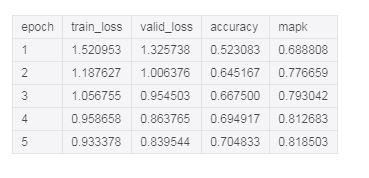# Add MAPK metric to fast-ai library

Hi all,

Kaggle has some competitions that use MAPK as evaluation metric(whale identification,doodle recognition…etc), but currently fast-ai metric only supports top-k accuracy. I am wondering if a PR to add MAPK is needed?

According to this poston Kaggle, I implemented the MAPK and tested on fast-ai Cifar10 dataset.

The idea is simple,

1. calculate single_prediction MAPK value
If targs has batch size (m, classes), then single_pred has size (1,classes)

2. take average of the single_prediction MAPK of the batch, fast-ai library will take care of the whole validation set.

def mapk(preds,targs,k=5):
batch_pred = preds.sort(descending=True) #batch_size * classes

def single_map(pred,label,k=5):
try:
return 1/ ((pred[:k] == label).nonzero().item()+1) #scalar division
except ValueError:
return 0.0

Here are some test result:

By default, it calculates MAP5You can also use partial(mapk,k) to change it to desired MAPk metric.
Here is an example of MAP1, it should match accuracy since it is calculating MAP1

Hi,

I used the same map@k function but getting the following error while training:

in mapk(targs, preds, k)
1 def mapk(targs,preds,k=5):
2 batch_pred = preds.sort(descending=True) #batch_size * classes
4
5 def single_map(label,pred,k=5):

in (.0)
1 def mapk(targs,preds,k=5):
2 batch_pred = preds.sort(descending=True) #batch_size * classes